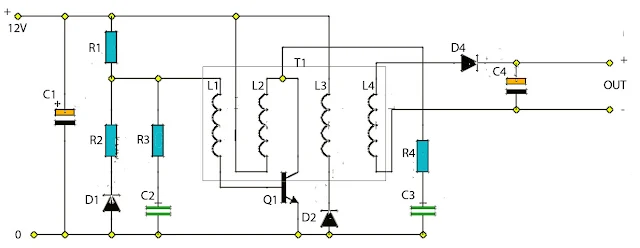# Portable Battery Charger Circuit

This circuit was designed to charge Nicad battery packs in the range of 4.8 to 15.6 V from a convenient remote power source , such as automobile battery. When power is first applied to the circuit applied to the circuit , a small bias current supplied by R1 via winding L1 , starts to turn on the transistor TR1.

### Portable Battery Charger Circuit DiagramThis forces a voltage across L2 and the positive feedback given by the coupling of L1 and L2 causes the transistor to turn hard on , applying the full supply across L2. The base drive voltage induced across L1 makes the junction the necessary base current to hold Q1 on.
Component List

Resistor
R1 = 1M
R2 = 120R
R3 = 10R
R4 = 39R

Capacitor
C1 = 100uF 25V
C2 = 0.01uF
C3 = 4700pF
C4 = 100uF 25V

Diode
D1 = 1N4148
D2 = BYV27-5
D3 = BYV27-5

Transistor
Q1 = ZTX650

Transformer
L1 = 12T 36awg
L2 = 13T 36awg
L3 = 20T 30awg
L4 = 40T 30awg
Core FX3437 with gap of 0.08mm
Former DT2492